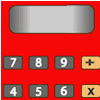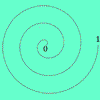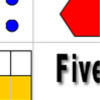More Number Fluency Tasks Upper Primary

These tasks will help children become more fluent in the context of number and calculation.  They supplement the activities in our Fluency Feature.  The first two are games and so could be revisited many times, whereas the remaining activities are one-off challenges.Forgot the Numbers

Age 7 to 11 Challenge Level:

On my calculator I divided one whole number by another whole number and got the answer 3.125. If the numbers are both under 50, what are they?Spiralling Decimals

Age 7 to 14 Challenge Level:

Take turns to place a decimal number on the spiral. Can you get three consecutive numbers?Number Match

Age 5 to 11 Challenge Level:

A task which depends on members of the group noticing the needs of others and responding.I'm Eight

Age 5 to 11 Challenge Level:

Find a great variety of ways of asking questions which make 8.Consecutive Numbers

Age 7 to 14 Challenge Level:

An investigation involving adding and subtracting sets of consecutive numbers. Lots to find out, lots to explore.Three Dice

Age 7 to 11 Challenge Level:

Investigate the sum of the numbers on the top and bottom faces of a line of three dice. What do you notice?Sealed Solution

Age 7 to 11 Challenge Level:

Ten cards are put into five envelopes so that there are two cards in each envelope. The sum of the numbers inside it is written on each envelope. What numbers could be inside the envelopes?Reach 100

Age 7 to 14 Challenge Level:

Choose four different digits from 1-9 and put one in each box so that the resulting four two-digit numbers add to a total of 100.